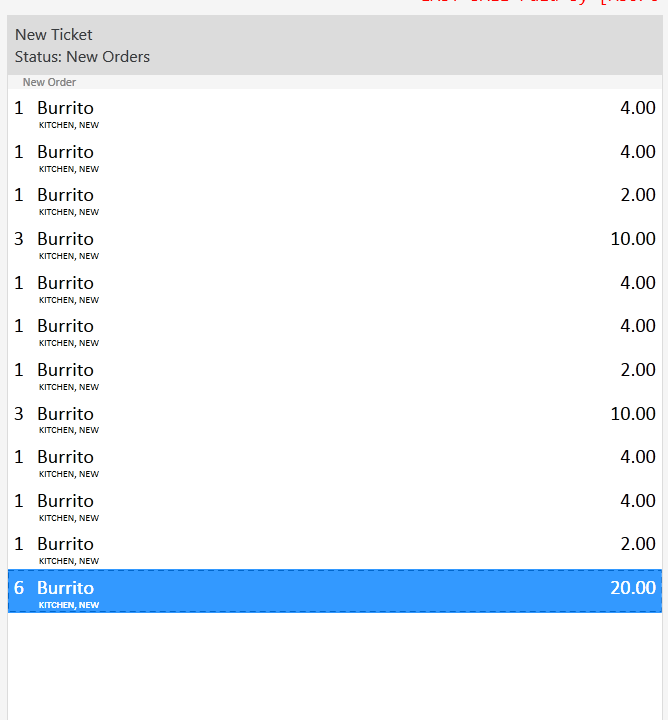# Formula driving me crazy!

I have a formula:

``````[=TN('{TOTAL PRICE}')-(TN('{PRICE}')*round down(TN('{TICKET QUANTITY SUM:Kaiser 600ml}')/3))+round down(TN('{TICKET QUANTITY SUM:Kaiser 600ml}')/3)]
``````

That calculates a new price for a specific promotional item.

This forula works fine in a `ShowMessage` action, but work horrible in a `ChangePrice` Action… Seems like `{TOTAL PRICE}` has a complete different value in the two actions.

what could be my error?

Thanks!!!

G.

What’s 19*5?

Try just {QUANTITY} instead of {TICKET QUANTITY SUM}

When you use quantity button vs seperate orders it adds formula then multiplies by qty.

I did not understand you…

Sorry.

g.

The way quantity works I different when you press 5 and then product. Vs pressing product 5 times. try replacing {TICKET QUANTITY SUM:X} with just {QUANTITY}

mmmmmmmmmmmmmmmmmmmmmmmmmmmmm let me see…

why in the messagebox {TICKET QUANTITY SUM:X} works, and why in the action not… that has nothing to do with {QUANTITY}, and the problem seem to be in the {PRICE}…

but I see your point, so I will try with {QUANTITY} instead of {…SUM:X…}

G.

I both cases

``````[=TN('{TOTAL PRICE}')-(TN('{PRICE}')*round down(TN('{QUANTITY}')/3))+round down(TN('{QUANTITY}')/3)]
``````

and

``````[=TN('{TOTAL PRICE}')-(TN('{PRICE}')*round down(TN('{TICKET QUANTITY SUM:Kaiser 600ml}')/3))+round down(TN('{TICKET QUANTITY SUM:Kaiser 600ml}')/3)]
``````

the formula works great in the `ShowMessage Action`, but throws a horrible result in the `Update Order Action`. and I think that the problem is with the `{TOTAL PRICE}` Tag… I cannot figure out why…

`{TOTAL PRICE}`should be`{PRICE} x {QUANTITY}`??
`{TOTAL AMOUNT}` does not work either, seems like all TOTAL for that line have a strange value, much mor higher that it should be.

G.

OK!!! I figured it out…

When you call the `UPDATE ORDER` action with new price, It automatically calculates multiplies the new price by `QUANTITY`… so thats why I was getting a strange price… I was beeing multiplied again…

G.

Here is what I have working so far:

This works with 1 exception if you add the beers by pressing Product, Product, Product and the third product goes on ticket as 2.00 directly after if you press 3 + Product it adds as 6.00

As long as you do not envoke the {TICKET QUANTITY SUM:X) Custom constraint directly before using {NUMBERPAD} + Product it works correctly.

This is kind of strange behavior it does not make sense to me. I can kind of see what it is doing… I just have not figured out a constraint to prevent it.

Here are the rules:

Here is output showing both behaviors… notice after the 2.00 beer and after the 4.00 beer quantity selections.

OMG I FIGURED IT OUT!!! Keep same rules as I just showed but MAKE SURE you sort them so the {QUANTITY} Rule comes After the {TICKET QUANTITY SUM:X} Rule

THe beautiful results…I never realized that the actual order the rules are listed in the Rules Menu also affect the order they fire!!!

I guess I should have known this it only makes sense it would work that way.

1 Like

GREAT JOB!!!

I was getting close, but not perfect… my formula was having strange result with large numbers since it included products that were alredy with promotinal price!

CONGRATULATIONS!!!and THNAK YOU VERY MUCH FOR HELPING ME OUT HERE!!!

G.

Dear @Jesse, did you try your method with:

2 times 2 burritos?
4 burritos?
5 burritos?
7 burritos?

I my case if a table with 12 people sits down, they will for sure ask for at least 4 beers at once…

so the best formula I came up so far is:

``````[=(TN('{TOTAL AMOUNT}')-(TN('{PRICE}')*round down(TN('{QUANTITY}')/3))+round down(TN('{QUANTITY}')/3))/TN('{QUANTITY}')]
``````

This are the rules:

The results are:

1 Like

So its working for you now? And no I did not. But looks like you have it tweaked and working better unless im reading it wrong… this little exercise has fried my brain lol. Its like we are trying to figure out Pi by ordering beers and burritos